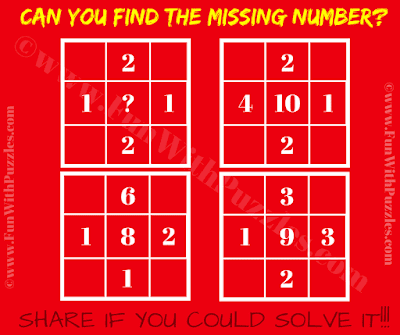It is Brain Twister Maths Puzzle in which you have to find the missing number which will replace the question mark. Here in this Maths Puzzle, there is 5 number given in four different squares. Number in each square relates to each other Mathematically. Can you decode this hidden Maths relationship among these five number and then find the value of the missing number?Can you find the missing number?

The answer to this "Maths Brain Twister Puzzle", can be viewed by clicking on the button. Please do give your best try before looking at the answer.